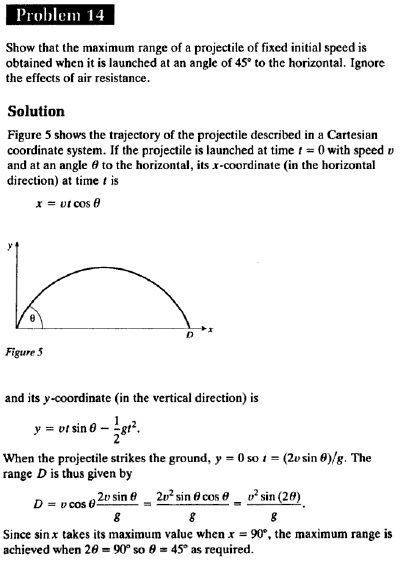# Maximum range of a projectile when launched at 45°(Physics by example, Rees, pr. 14)In this solution the t in the y-coordinate equation is substituted using the x-coordinate equation and ultimately leads to the answer.

My questions:
1. Why don't I get the same answer when I substitute the v or v and t instead?
2. How am I supposed to know to substitute t in this example and not v?

## Answers and Replies

Delphi51
Homework Helper

I don't see how you can get it by keeping the t. You don't know t, so how can you tell the maximum value of x that way? I get
x = gt²/(2*tanΘ)
and can't tell what combination of t and Θ provide the maximum x.
It would be good to see your calc.

There is no need to eliminate the v; it is a constant. But you must eliminate the variable t.

Well, the problem is quite easy to approach.
Range is given by u^2 sin(2a) / g, where a is the projection angle.
Since -1<sin a<1, the max. value for a sine function = 1. This occurs when the angle is 90 degrees or .5pi radians.
So, for a fixed u:
2a = 90
a = 45 degrees.

I don't see how you can get it by keeping the t. You don't know t, so how can you tell the maximum value of x that way? I get
x = gt²/(2*tanΘ)
and can't tell what combination of t and Θ provide the maximum x.
It would be good to see your calc.

There is no need to eliminate the v; it is a constant. But you must eliminate the variable t.

Ok, now I understand. Thnx!